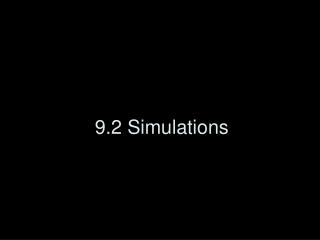DownloadDownload Presentation9.2 Simulations

# 9.2 Simulations

Download Presentation## 9.2 Simulations

- - - - - - - - - - - - - - - - - - - - - - - - - - - E N D - - - - - - - - - - - - - - - - - - - - - - - - - - -
##### Presentation Transcript

1. 9.2 Simulations

2. Simulations • We think of a model as a set of equations describing what we observe in a real-world experiment. • When we can’t even do the experiment (e.g., nuclear testing), the model can be considered a simulation.

3. Monte Carlo Simulations A Monte Carlo simulation is a probabilistic model involving an element of chance.

4. Genesis of Monte Carlo Simulations John(ny) von Neumann (1903-1957) • Game theory • Quantum mechanics • Economics • Cellular automata • Nuclear weapons • Computer science (“von Neumann bottleneck)

5. (Pseudo)Random Numbers • True randomness is rare (radioactive decay) • In practice, we mimic randomness with a deterministic process • Linear congruential method uses modulus (clock) arithmetic to generate a sequence r : r0 = 10 rn = (7 rn -1 + 1) mod 11, for n > 0

6. Linear Congruential Method • In general: r0 = seed rn = (multiplier *rn-1 + increment) mod modulus, for n > 0 • Much research is devoted to methods for making these sequences difficult to predict (e.g., for codebreaking) • Periodicity (length before repetition) should be as large as possible.

7. Different Ranges of Random Numbers • May want to restrict range of numbers, get floating-point values, etc. • Modulus tells us the maximum integer we’ll get. So we can divide by that max to get a floating-point value r in the interval [0,1]. • We can then rescale to a new min, max: • s = (max – min) * r + min